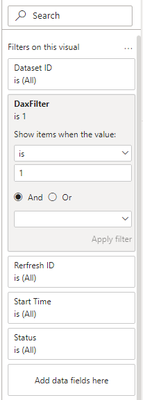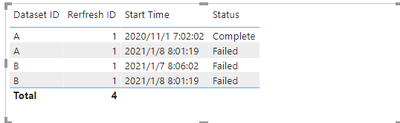cancel
Showing results for
Did you mean:## Filter Twice to Get the Table We Need

Scenario:
As shown in the following sample table, when we want to get all the values of the ID with the latest start time, we need to filter twice to get the result.
Ex: The Dataset ID (A) contains the latest Start Time. Now we want to get all rows which contain Dataset ID A. We can see Dataset ID A has two rows. If we only filter the latest Start Time, we can only get one row of A.
Therefore, we first get the dataset ID based on the latest start time, and then get all the values by filtering the previous obtained dataset ID(A).
If you want to get result in the example table below, you can use Power Query or Dax.

Table Used:
Here is my sample table. I want to display all rows which contain Dataset ID A (A has the latest Start Time: 2021/1/8 8:01:19 AM)Method 1: In Power Query
The operation steps are as follows:
1. We need to get the Dataset ID with the latest start time.

``MaxTime = Table.SelectRows(Source, each ([Start Time] = List.Max(Source[Start Time])))``2. We need to get all values in A not just the ones with newest Start Time. Therefore we need to filter the original table again by the Dataset ID we got in MaxTime.

``Table.SelectRows(Source, each ([Dataset ID] = List.Max(MaxTime[Dataset ID])))``

``````let
Source = Table.FromRows(Json.Document(Binary.Decompress(Binary.FromText("bdHNCoMwDAfwV5GehTb9sNqb07kP2BOIB8c8CI4Ntvdnaeu2gD30kD8/0pD0PatZzgCfFBI48DIrnQAHVVZfMO3GeZlubMh7tvPoD62HhRNyAxssVYSCA/DKS+2EibKdX+P1a1sMNLHhe/Xr2jzuz2V6T8HuMTDE2thXqoTtMCiILbyVTuqEPWBgiTXRilTfIwYlsXpdl0nYEwYVsWqdQSTs2V9BECzjImSZwOFkQDBkNky82drwAQ==", BinaryEncoding.Base64), Compression.Deflate)), let _t = ((type nullable text) meta [Serialized.Text = true]) in type table [#"Dataset ID" = _t, #"Rerfresh ID" = _t, #"Start Time" = _t, Status = _t]),
#"Changed Type" = Table.TransformColumnTypes(Source,{{"Dataset ID", type text}, {"Rerfresh ID", Int64.Type}, {"Start Time", type datetime}, {"Status", type text}}),
#"Filter Row" =
let
MaxTime = Table.SelectRows(Source, each ([Start Time] = List.Max(Source[Start Time])))
in
Table.SelectRows(Source, each ([Dataset ID] = List.Max(MaxTime[Dataset ID])))
in
#"Filter Row"``````

Result is as below.Extend:
If there are multiple IDs (Ex:A, B) with the same newest Start Time, the M Query above will only return to the Max ID with the List.Max function. We can try List.Contain function in M Query.

``````let
Source = Table.FromRows(Json.Document(Binary.Decompress(Binary.FromText("ddHLCsIwEAXQXylZC8nk0aTZ1db6AL+gdFGxi0JFQf8fJ03VQeMii1wOkzC3bVnJVgzwSCGBA3eZ8wI8FFl5xLTpx2k4s27VsnVAH2gDzL2QP7DCq4pQcABeBKm9MFHW470/vWyNgSZ2fl69p1bXy20aHsNsNxgYYm2cK1XCNhjkxObBSi91wm4xsMSaaEVq7g4DR6xe1mUSdo9BQaxa/iAS9hBaEATLuAjpEniuDAiGzM4/Tm3tq7Y//XZP", BinaryEncoding.Base64), Compression.Deflate)), let _t = ((type nullable text) meta [Serialized.Text = true]) in type table [#"Dataset ID" = _t, #"Rerfresh ID" = _t, #"Start Time" = _t, Status = _t]),
#"Changed Type" = Table.TransformColumnTypes(Source,{{"Dataset ID", type text}, {"Rerfresh ID", Int64.Type}, {"Start Time", type datetime}, {"Status", type text}}),
#"Filter Row" =
let
MaxTime = Table.SelectRows(Source, each ([Start Time] = List.Max(Source[Start Time])))
in
Table.SelectRows(#"Changed Type", each List.Contains(MaxTime[Dataset ID], [Dataset ID])),
#"Sorted Rows" = Table.Sort(#"Filter Row",{{"Dataset ID", Order.Ascending}})
in
#"Sorted Rows"``````

Result is as below.Method 2: In DAX
The operation steps are as follows:
1. We need to get the latest time first.
2. Then look up the dataset ID based on the latest time we got earlier
3. Finally, use IF function to return 1 when the Dataset ID equals to the dataset ID with the newest time, others will return 0.
4. Build a Table visualization, add this measure into the filter field of this visual and set this filter to show items when value = 1.Dax in Measure:

``````DaxFilter =
VAR _MaxTime =
MAXX ( ALL ( Table2 ), Table2[Start Time] )
VAR _DatasetID =
CALCULATE (
MAX ( Table2[Dataset ID] ),
FILTER ( ALL ( Table2 ), Table2[Start Time] = _MaxTime )
)
RETURN
IF ( MAX ( Table2[Dataset ID] ) = _DatasetID, 1, 0 )``````

Result is as below.Extend:
For the same error as the M Query , we need to update our Dax if there are more than one ID (Ex:A, B) with the same latest Start Time.
Dax in Measure:

``````DaxFilter2 =
VAR _MaxTime =
MAXX ( ALL ( 'Table2 (2)' ), 'Table2 (2)'[Start Time] )
VAR _DatasetID =
CALCULATETABLE(
VALUES('Table2 (2)'[Dataset ID]),
FILTER ( ALL('Table2 (2)') , 'Table2 (2)'[Start Time] = _MaxTime )
)
RETURN
IF ( MAX ( 'Table2 (2)'[Dataset ID] ) in _DatasetID, 1, 0 )``````

Result is as below.See attached file for details.

Author: Rico

Reviewer:  Kerry and Ula

Top Kudoed Posts
Latest Articles
Archives
Polls
What is your favorite Power BI feature release for September 2023?